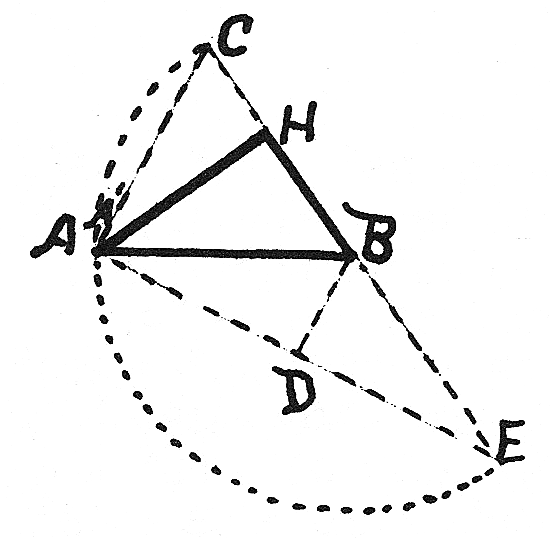#The Pythagorean Proposition: Algebraic Proofs, Proof #53

Math Lair Home > Source Material > The Pythagorean Proposition > Algebraic Proofs, Proof #53

### Fifty-ThreeFig. 51

An indirect algebraic proof, said to be due to the great Leibniz  (1646-1716).

If (1) HA² + HB²= AB², then (2) HA² = AB² - HB², whence (3) HA² = (AB + HB)
(AB - HB).

Take BE and BC each equal to AB, and from B as center describe the semicircle CA'E.  Join AE and AC, and draw BD perp. to AE. Now (4) HE = AB + HB, and (5) HC = AB - HB. (4) × (5) gives HE × HC = HA², which is true only when triangles AHC and EHA are similar.

∴ (6) angle CAH = angle AEH, and so (7) HC
: HA = HA : HE; since angle HAC = angle E, then angle CAH = angle EAH.  ∴ angle AEH + angle EAH = 90° and angle CAH + angle EAH = 90°.  ∴ angle EAC = 90°.  ∴ vertex A lies on the semicircle, or A coincides with A'.  ∴ EAC is inscribed in a semicircle and is a rt. angle. Since equation (1) leads through the data de­rived from it to a rt triangle, then starting with such a triangle and reversing the argument we arrive at h² = a² + b².

a. See Versluys, p. 61, fig. 65, as given by von Leibniz.